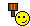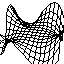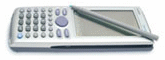# Math Function in CP300 !

10 replies to this topic

### #1PJay

PJay

Casio Freak

•• Members
•• 260 posts
• Gender:Male

• Calculators:
Casio fx-991ES Plus

Posted 05 December 2003 - 02:41 PM

Hi, Can CP300 solve this question ?If "f(2x-3)=x^2-1" then "f(x)=?"### #2BiTwhise

BiTwhise

Casio Overgod

•• [Legends]
•• 627 posts
• Gender:Male
• Location:Guildford, Surry, UK
• Interests:Programming, games, consoles, martial arts

• Calculators:

Posted 08 December 2003 - 12:55 AM

this is basically two simultanious eqations..

Solve the part inside the brackets for 'x'
Calling the function input 'N', you have:

f(N) = x^2-1
and
N=2x-3

Solve N=2x-3, for x, and you get
x=(N+3)/2

Now you substitute this into your function definition
f(N) = ((N+3)/2)^2-1 = (N+1)(N+5)/4

There you have your function defined as f(N)..

If you wondered if the CP would do this directly with some magic function.. there is a slight possibility that you can do it in the sequence app perhaps :/
Haven't explored those terretories too much, so I can't really help

### #3Lovecasio

Lovecasio

Casio Freak

•• Members
•• 242 posts
• Location:Hochiminh city Vietnam
• Interests:Organic chemistry.<br />Pharmacy

• Calculators:
fx 570 MS, Casio AFX 2.0+, ClassPad 300

Posted 08 December 2003 - 01:23 AM

I have other solution:
Define f(x)=2x-3
Define g(x)=x^2-1
I use these command because as you write, f(2x+3)=x^2-1, so for every value of x, we have f(a) and g(a). Two side are equal. To get the equation of f(x), use:
sequence({f(1),f(2),f(3)},{g(1),g(2),g(3)})
I use 3 factor because the degree of right side is two (x^2-1). This will return f(x). (As I calculate by ClassPad, f(x)=x^2/4+3x/2+5/4.
Is it right?(You can also make program to solve)

### #4BiTwhise

BiTwhise

Casio Overgod

•• [Legends]
•• 627 posts
• Gender:Male
• Location:Guildford, Surry, UK
• Interests:Programming, games, consoles, martial arts

• Calculators:

Posted 08 December 2003 - 02:54 AM

That's the same solution as I got, yes### #5maurice1979

maurice1979

Casio Fan

•• Members
•• 40 posts
• Location:The netherlands - Dutch people

• Calculators:
CP300, Fx8.... fraction sumtin', old thing with dust, it still works, with the original battery

Posted 08 December 2003 - 12:50 PM

I found another jugde() "won't" solve
Judge(inf/inf =1) it gives undefined the awnser could be false, BUT the awser can not be defined.
At least I think so.sooooo....
when my CP give Undefined, is it safe to say the awser is false???

### #6BiTwhise

BiTwhise

Casio Overgod

•• [Legends]
•• 627 posts
• Gender:Male
• Location:Guildford, Surry, UK
• Interests:Programming, games, consoles, martial arts

• Calculators:

Posted 08 December 2003 - 02:58 PM

Nopes...

inf/inf = undefined...

It could be = 1.. so it's not false.. and it could be equal to anything else, so it's not true..

### #7Lovecasio

Lovecasio

Casio Freak

•• Members
•• 242 posts
• Location:Hochiminh city Vietnam
• Interests:Organic chemistry.<br />Pharmacy

• Calculators:
fx 570 MS, Casio AFX 2.0+, ClassPad 300

Posted 09 December 2003 - 03:59 PM

The limit of 1/x as x approaches 0 is infinity. However, ClassPad gives "Undefined". What do you think?

### #8BiTwhise

BiTwhise

Casio Overgod

•• [Legends]
•• 627 posts
• Gender:Male
• Location:Guildford, Surry, UK
• Interests:Programming, games, consoles, martial arts

• Calculators:

Posted 09 December 2003 - 04:52 PM

the limit of 1/x as x approaches 0 is undefined, unless you define from which direction you take the limit, in which case it would be + or - inf

on the classpad you would do:

lim(1/x,x,0,1) <- take the limit from the positiv side
gives:
oo

lim(1/x,x,0,-1) <- take the limit from the negative side
gives:
-oo

### #9Lovecasio

Lovecasio

Casio Freak

•• Members
•• 242 posts
• Location:Hochiminh city Vietnam
• Interests:Organic chemistry.<br />Pharmacy

• Calculators:
fx 570 MS, Casio AFX 2.0+, ClassPad 300

Posted 10 December 2003 - 12:45 AM

As I know, if lim(f(x))=0 and f(x)<>0 with all x near enough a then lim(1/f(x))=oo. In this case, we have lim(x,x,0)=0 ,but f(x) can equal 0 as x approaches 0.
Well, I have just study about limitation at highschool, grade 11. There are alot of things I don't know. Please tell me if I am wrong.I agree with you. Limit of f(x) is defined if and only if lim(f(x),-1)=lim(f(x),1)=lim(f(x))=L
Thanks.### #10Overlord

Overlord

Casio Technician

•• Moderator
•• 355 posts
• Gender:Male
• Location:Brussels - Belgium
• Interests:Math Researcher

• Calculators:
My head - C300 OS 3.00 - G100 Rom 1.02 - G65 - G60 - G25

Posted 10 December 2003 - 08:37 PM

" if lim(f(x))=0 and f(x)<>0 with all x near enough a then lim(1/f(x))=oo "

With f(x) = x and limit at x=0:

lim(f(x))=0
f(x)=/=0 for all x =/= 0

but lim(1/f(x)) =/= oo (the limit is undefined)

"In this case, we have lim(x,x,0)=0 ,but f(x) can equal 0 as x approaches 0."

lim = 0 but f(x)=x =/=0 when x approaches 0 (i suppose that approach => not equal)

other example :

f(x) = x for x=/=0, f(x) undefined for x=0

limit at x=0 : lim (f(x)) = 0 = right limit at 0 = left limit at 0
f(x) never = 0

limit at x=0 : lim (1/f(x)) = Undefined

lim 1/f = +oo (-oo)
if f is also positive (negative) before and after the limit point (no signum change)

### #11betoe

betoe

UCF Spanish Translator

•• [Legends]
•• 846 posts
• Gender:Male
• Interests:Electronics, SW development, automotive. Swim->bike->run

• Calculators:

Posted 14 December 2003 - 05:16 AM

Another math doubt:
I want to calculate the fourier coefficients. I do it with the CP when i have all the values of each variable (k,n,N), but when i dont have the N value and i must do it like formula, i dont know how...

I do this on my class and i want to make it on the CP:
ck= fourier coefficient= A/N {sum(n=0 to L-1) [e^(-i*2pi*k/N)^n] }

I hope that somebody can understand me and help me(i'm not good explaining maths in english, i dont know well the math terms in this language).

#### 0 user(s) are reading this topic

0 members, 0 guests, 0 anonymous users